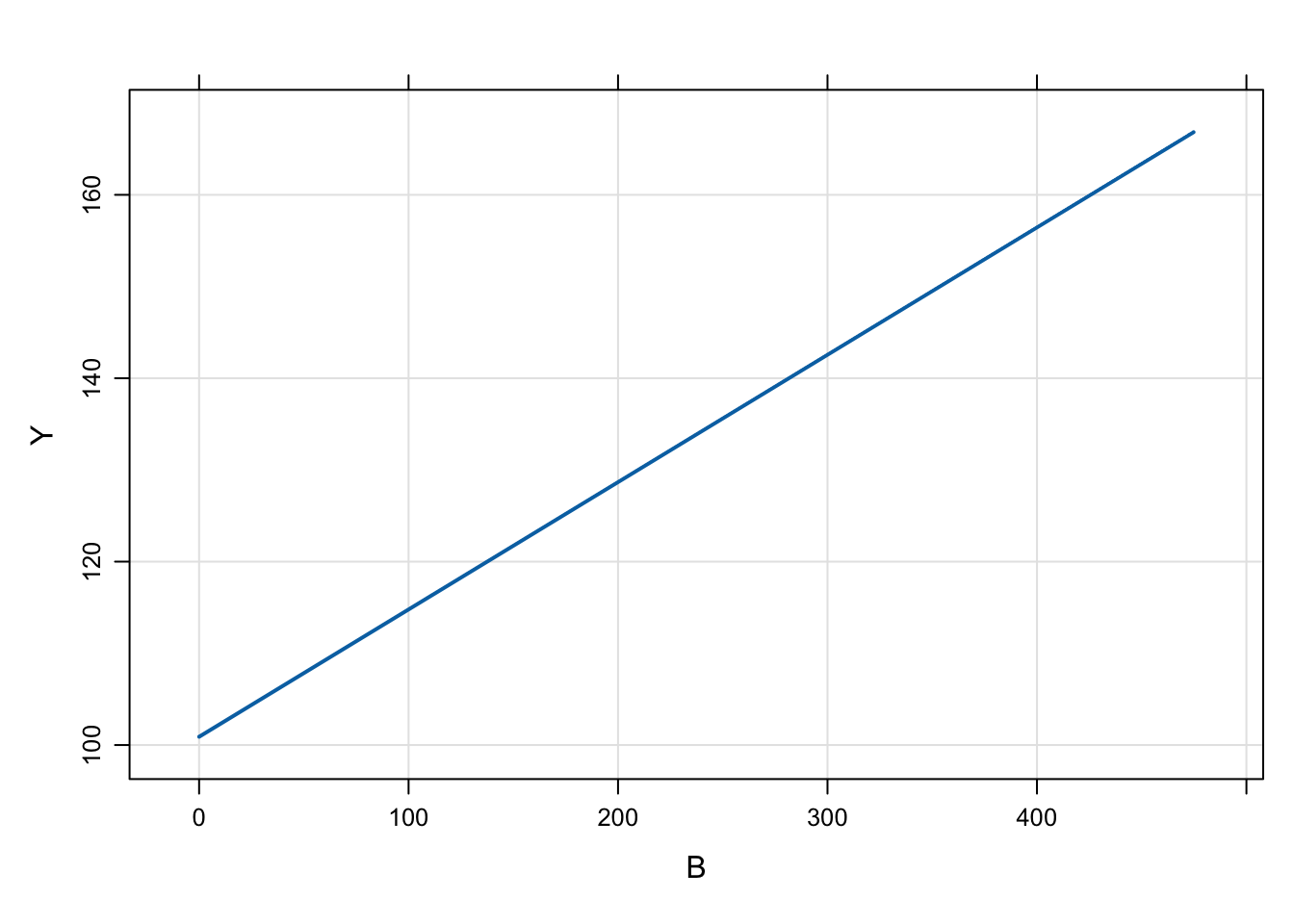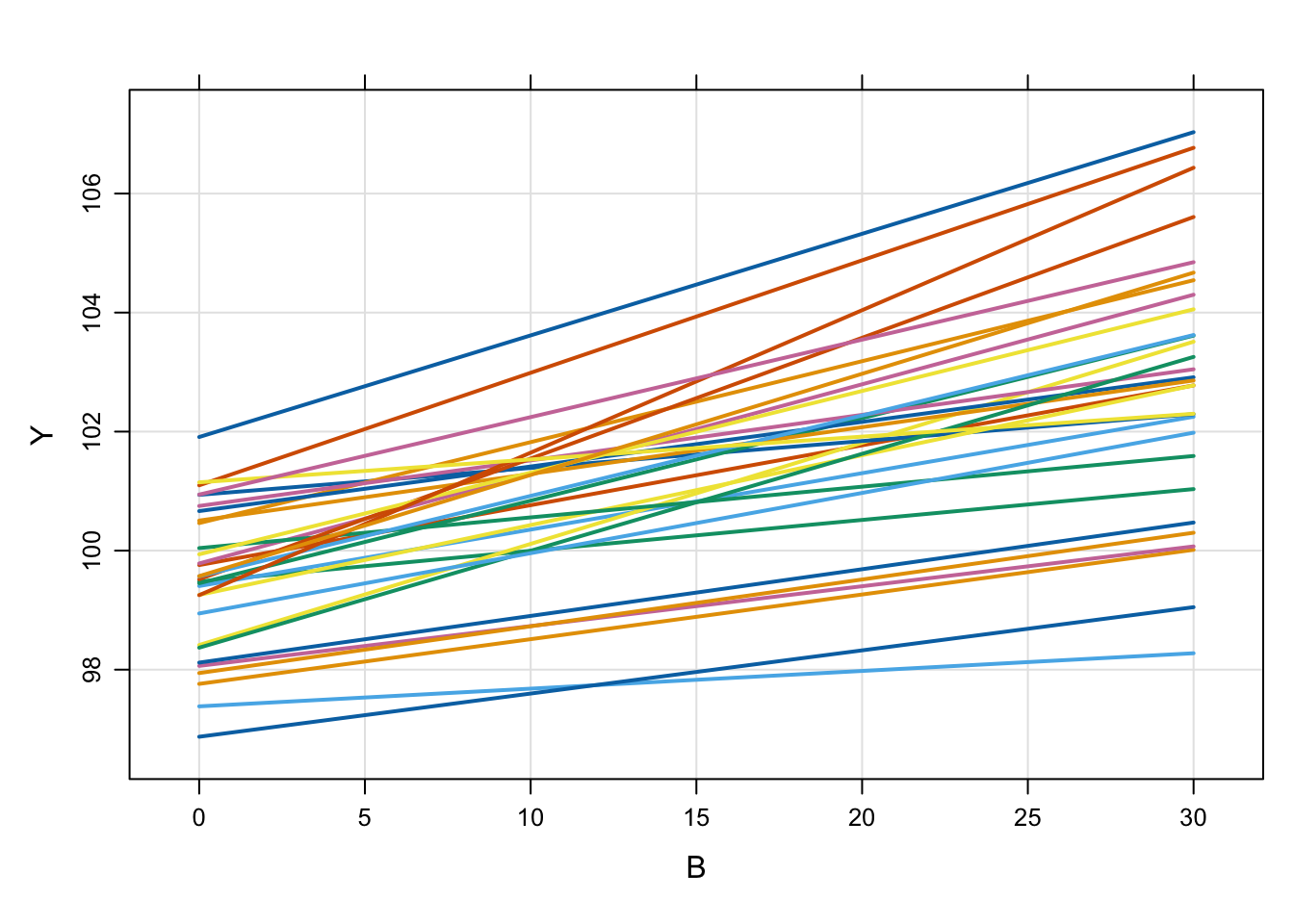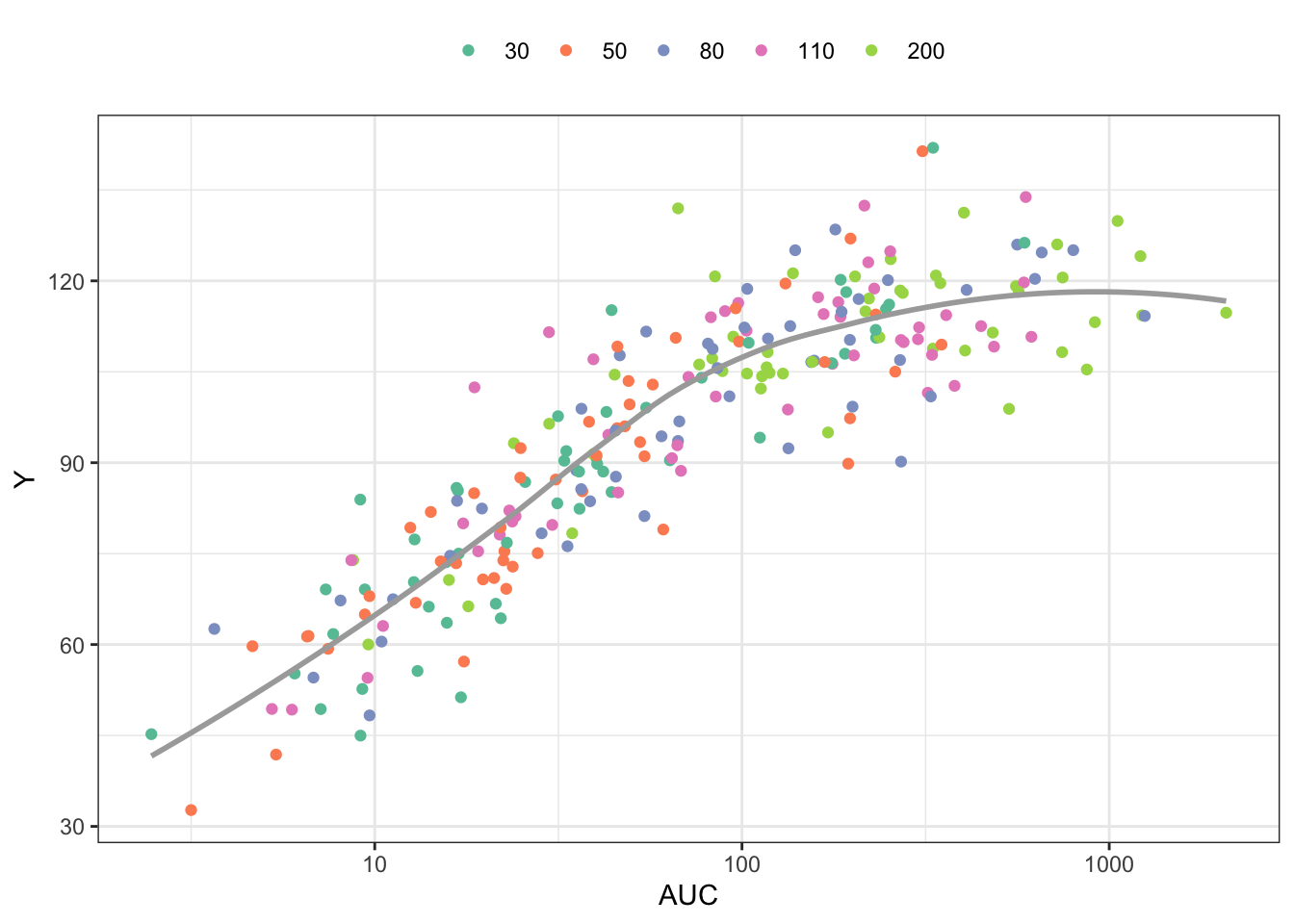# Models without compartments

Introducing a new model block (`\$PRED`) that lets you write models with analytical solutions.

pred
model specification
Author

Kyle Baron

Published

January 1, 2018

# 1 Introduction

This post introduces a new formal code block for writing models where there are no compartments. The block is named after the analogous NONMEM block called `\$PRED`. This functionality has always been possible with mrgsolve, but only now is there a code block dedicated to these models. Also, a relaxed set of data set constraints have been put in place when these types of models are invoked.

# 2 Example model

As a most-basic model, we look at the `pred1` model in `modlib()`

``````library(mrgsolve)
options(mrgsolve.soloc="build")

The model code is

``````\$PROB
An example model expressed in closed form
\$PARAM B = -1, beta0 = 100, beta1 = 0.1
\$OMEGA 2 0.3
\$PRED
double beta0i = beta0 + ETA(1);
double beta1i = beta1*exp(ETA(2));
capture Y = beta0i + beta1i*B;``````

This is a random-intercept, random slope linear model. Like other models in mrgsolve, you can write parameters (`\$PARAM`), and random effects (`\$OMEGA`). But the model is actually written in `\$PRED`.

When mrgsolve finds `\$PRED`, it will generate an error if it also finds `\$MAIN`, `\$TABLE`, or `\$ODE`. However, the code that gets entered into `\$PRED` would function exactly as if you put it in `\$TABLE`.

In the example model, the response is a function of the parameter `B`, so we’ll generate an input data set with some values of `B`

``````library(dplyr)
data <- tibble(ID = 1, B = exp(rnorm(100, 0,2)))

``````# A tibble: 6 × 2
ID       B
<dbl>   <dbl>
1     1   3.76
2     1 196.
3     1   2.90
4     1   0.168
5     1   0.278
6     1   0.773``````
``````out <- mrgsim_d(mod,data,carry.out="B")

plot(out, Y~B)``````Like other models, we can simulate from a population

``````library(purrr)
set.seed(223)
df <- map_df(1:30, function(i) tibble(ID = i, B = seq(0,30,1)))

``````# A tibble: 6 × 2
ID     B
<int> <dbl>
1     1     0
2     1     1
3     1     2
4     1     3
5     1     4
6     1     5``````
``````mod %>%
data_set(df) %>%
mrgsim(carry.out="B") %>%
plot(Y ~ B)``````# 3 PK/PD Model

Here is an implementation of a PK/PD model using `\$PRED`

In this model

• Calculate `CL` as a function of `WT` and a random effect
• Derive `AUC` from `CL` and `DOSE`
• The response (`Y`) is a calculated from `AUC` and the Emax model parameters
``````code <- '
\$PARAM TVCL = 1, WT = 70, AUC50 = 20, DOSE = 100, E0 = 35, EMAX = 2.4

\$OMEGA 1

\$SIGMA 100

\$PRED
double CL = TVCL*pow(WT/70,0.75)*exp(ETA(1));
capture AUC = DOSE/CL;
capture Y = E0*(1+EMAX*AUC/(AUC50+AUC))+EPS(1);
'``````
``mod <- mcode_cache("pkpd", code)``
``Loading model from cache.``

To simulate, look at 50 subjects at each of 5 doses

``````data <-
expand.idata(DOSE = c(30,50,80,110,200),ID = 1:50) %>%
mutate(WT = exp(rnorm(n(),log(80),1)))

``````  ID DOSE        WT
1  1   30  59.13254
2  2   50 317.32739
3  3   80 242.15746
4  4  110 170.78136
5  5  200 248.18054
6  6   30  51.22012``````
``````out <- mrgsim_d(mod,data,carry.out="WT,DOSE") %>% as.data.frame

``````  ID time        WT DOSE       AUC         Y
1  1    0  59.13254   30 231.90852 110.61330
2  2    0 317.32739   50  36.76051  85.27834
3  3    0 242.15746   80  36.54808  98.90407
4  4    0 170.78136  110  23.68354  80.29131
5  5    0 248.18054  200 331.10229 108.81926
6  6    0  51.22012   30 251.58373 116.09649``````

Plot the response (`Y`) versus `AUC`, colored by dose

``````library(ggplot2)

ggplot(out, aes(AUC,Y,col =factor(DOSE))) +
geom_point() +
scale_x_continuous(trans = "log", breaks = 10^seq(-4,4)) +
geom_smooth(aes(AUC,Y),se = FALSE,col="darkgrey") + theme_bw() +
scale_color_brewer(palette = "Set2", name = "") +
theme(legend.position = "top")``````
```geom_smooth()` using method = 'loess' and formula = 'y ~ x'``